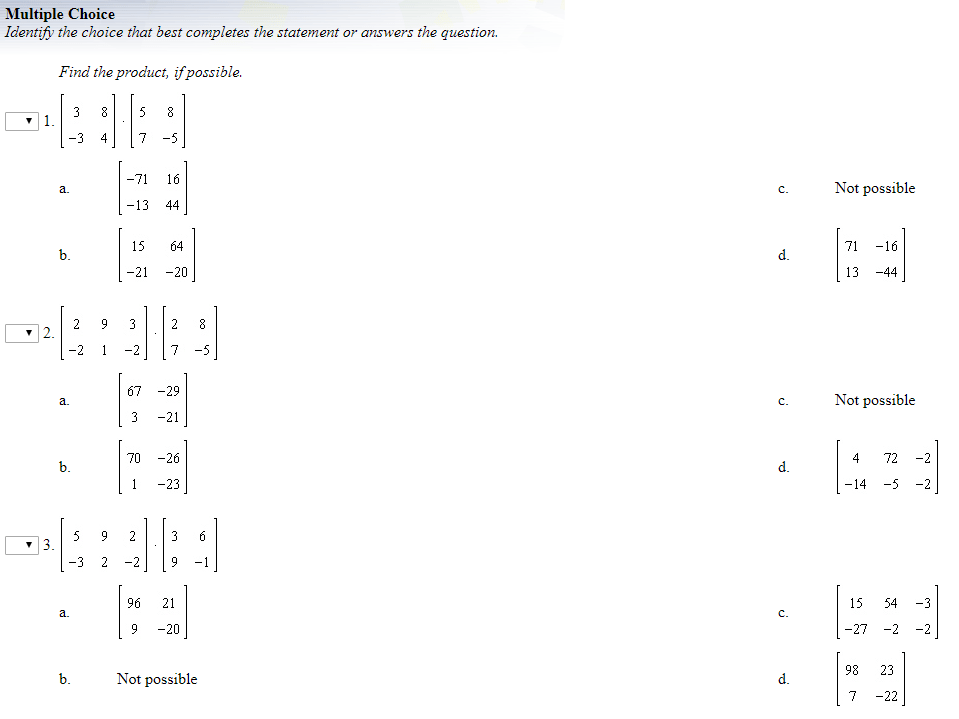# Multiplying MatricesAnswers (D, C, B, C, A, A  Remember: Multiplication of matrices is possible if and only if the number of columns in the first matrix is equal to the number of rows in the second matrix. The commutative property does not hold for matrices but the distributive does.)

(source)